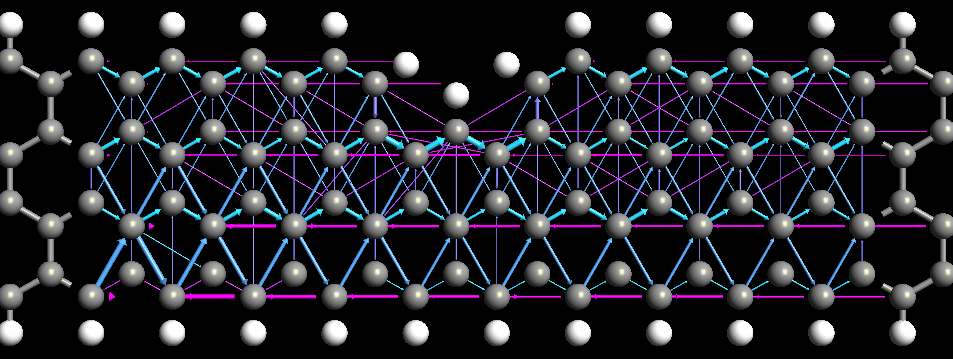# TransmissionPathways¶

class TransmissionPathways(configuration=None, energy=None, kpoints=None, contributions=None, self_energy_calculator=None, energy_zero_parameter=None, infinitesimal=None)

Class for representing the Transmission Pathways for a given device configuration and calculator.

Parameters: configuration (DeviceConfiguration) – The device configuration with attached calculator for which the transmission pathways should be calculated. energy (PhysicalQuantity of type energy) – The energy for which the transmission pathway should be calculated. Default: 0.0*eV kpoints (sequence (size 3) of int | MonkhorstPackGrid | KpointDensity) – The k-points for which the transmission pathways should be calculated. Note that the k-points must be in the xy-plane. Default: MonkhorstPackGrid(na, nb) where (na, nb) is the sampling used for the self-consistent calculation. contributions (Left | Right) – The transmission pathways contributions from the left or right electrode. Default: Left self_energy_calculator (DirectSelfEnergy | RecursionSelfEnergy | SparseRecursionSelfEnergy | KrylovSelfEnergy) – The self energy calculator to be used for the transmission pathways. Default: RecursionSelfEnergy(storage_strategy=NoStorage()) energy_zero_parameter (AverageFermiLevel | AbsoluteEnergy.) – Specifies the choice for the energy zero. Default: AverageFermiLevel infinitesimal (PhysicalQuantity of type energy.) – Small positive energy, used to move the transmission calculation away from the real axis. This is only relevant for recursion-style self-energy calculators. Default: 1.0e-6*eV
electrodeFermiLevels()
Returns: The left and right electrodes Fermi levels in absolute energies. PhysicalQuantity
energy()
Returns: The energy used in this transmission pathways. PhysicalQuantity of type energy
energyZero()
Returns: The energy zero value. PhysicalQuantity of type energy
evaluate(spin=None)

Return the atom transmission pathways.

Parameters: spin (Spin.Up | Spin.Down | Spin.Sum | Spin.X | Spin.Y | Spin.Z | Spin.UpUp | Spin.DownDown | Spin.RealUpDown | Spin.ImagUpDown) – The spin used in the analysis Default: Spin.Sum A list with transmission pathway data for the give spin type. Each entry in the list contains the data (atom index i, neighbour atom index j, repetitions, t_ij), where repetitions=(n_A, n_B, n_C) is the repetitions of the neighbour atom in directions A, B, C, and t_ij is the local bond transmission from i to j. list
infinitesimal()
Returns: The infinitesimal used for calculating the transmission pathways. PhysicalQuantity of type energy
metatext()
Returns: The metatext of the object or None if no metatext is present. str | unicode | None
nlprint(stream=None)

Print a string containing an ASCII table useful for plotting the AnalysisSpin object.

Parameters: stream (python stream) – The stream the table should be written to. Default: NLPrintLogger()
setMetatext(metatext)

Set a given metatext string on the object.

Parameters: metatext (str | unicode | None) – The metatext string that should be set. A value of “None” can be given to remove the current metatext.

## Usage Examples¶

Calculate the TransmissionPathways for a device configuration, print out the result, and save them for visualization with QuantumATK:

pathways = TransmissionPathways(device_configuration)
nlprint(pathways)
nlsave('pathways.nc',pathways)Fig. 177 Spin up component of the transmission pathways of a graphene ribbon with a wedge. Arrows point in the direction of the pathways.The magnitude of the pathway is illustrated by the volume of the arrow and the color shows the direction, i.e. right point arrows are blue and left pointing arrows violet.

Check, that the pathways sum up to the correct transmission spectrum:

pathways = TransmissionPathways(device_configuration)
border_atom = len(device_configuration.elements())/2
print 'transmission coefficient ',
print pathways._transmissionCoefficient(border_atom=border_atom)